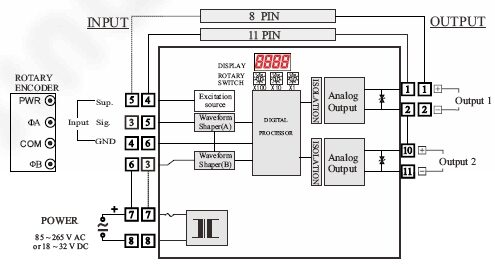# NFDC (Pulse,Frequency / DC) ROTARY SWITCH

· Function(기능)
Pulse,Frequency / DC converter
· Input(입력)
open-C / dry-C / Voltage/ Current
· Output(출력)
mA,V / dc 2-outputs
· Other Spec.(기타)
Pulse, Frequency
###### 제품 상세정보

□ Function & Features
– Frequency or Pulse inputs
– 10-position rotary switch : ( x100, x10, x1 ) x Rate(Hz)
– Rotary Switch Type :
The Input range is set by using a combination of Rate(Hz) and the rotary switches.
The rotary selector value is multiplied by the multiplication factor set on Rate(Hz)
The minimum full-scale value for the input is : 001 x 0.0001 = 0.0001 Hz
The maximum full-scale value for the input is : 999 x 1000 = 999000 Hz
*** ( Input of 50KHz or more is an order specification. )
– Four-way isolation (input/output1/output2/power)
– Protection Input and output TVS diode
– Analog One or Two outputs (independent output module)
– Universal power input
– Power fuse (240V/0.12A)

※ROTARY SWITCH

Input
The Input range is set by using a combination of Rate(Hz) and the rotary switches.
The rotary selector value is multiplied by the multiplication factor set on RATE(Hz)

For Example:
The input frequency full-scale value is 563Hz.
The 100’s selector is set to 5, the 10’s selector is set to 6 and the 1’s selector
is set to 3.
The multiplier is then set to 5(x1) (563 x 1 = 563Hz).
The minimum full-scale value for the input is : 001 x 0.0001 = 0.0001 Hz
The maximum full-scale value for the input is : 999 x 1000 = 999000 Hz
*** ( Input of 50KHz or more is an order specification. )

Calculation of input frequency : (( N x100 ) + ( N x10 ) + ( N x1 ) ) x (6:x10 Rate(Hz))
(( 1 x100 ) + ( 2 x10 ) + ( 3 x1 ) ) x ( 10 ) = 123 x 10 = 1230 Hz
(( 1 x100 ) + ( 2 x10 ) + ( 3 x1 ) ) x ( 0.1 ) = 123 x 0.1 = 12.3 Hz

■ INPUT SPECIFICATIONS

Excitation : 12V DC ±2V @30mA

Open Collector
Max. frequency : 100 Khz
Sensing : Approx. 12V DC @2.5 mA
ON/OFF Level : >OFF ( >2.5㏀/6V ), ON ( <1.6㏀/4V )
– DC Voltage Pulse
Max. frequency : 100 KHz
Waveform : Square or Sine
Input impedance : 10 ㏀ min.
Input Amplitude : 2~50Vp-p
Detecting Level : 12V pulse : Low(off) < 4V – 6V < High(on)
5V pulse : Low(off) < 1V – 3V < High(on)
– AC Voltage Pulse
Max. frequency : 100 KHz
Waveform : Sine
Input impedance : 10 ㏀ min.
Input Amplitude :0.1~100Vp-p (±50V max.)
– Two-wire Current Pulse
Max. frequency : 100 KHz
Input Resistance : 250 Ω
Input Range : 0 ~ 25mA
Detecting Level : Low(off) < 4mA – 12mA < High(on)

– 10-position rotary switch : (X100,X10,X1) x (X)rate
– Frequency Range : 0.0001 to 999000 Hz (*** Input of 50KHz or more is an order specification. )

■ ANALOG OUTPUT :
– DC Current : 0(4)~20 mA DC max. ( Load resistance : 600Ω max. )
– DC Voltage : -10V min. ~ +10V DC max. ( Load Resistance : 10 KΩ or more )
– 2-Wire Transmitter(4~20mA DC), supply out voltage (9 V ~ 35 V DC)
– Easy Calibration of the Gain (Max. ±5% of F.S) and Offset(Max. ±5% of F.S).